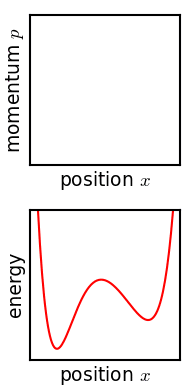# 1.4: Phase Space

•• Mark Tuckerman
• New York University
$$\newcommand{\vecs}{\overset { \rightharpoonup} {\mathbf{#1}} }$$ $$\newcommand{\vecd}{\overset{-\!-\!\rightharpoonup}{\vphantom{a}\smash {#1}}}$$$$\newcommand{\id}{\mathrm{id}}$$ $$\newcommand{\Span}{\mathrm{span}}$$ $$\newcommand{\kernel}{\mathrm{null}\,}$$ $$\newcommand{\range}{\mathrm{range}\,}$$ $$\newcommand{\RealPart}{\mathrm{Re}}$$ $$\newcommand{\ImaginaryPart}{\mathrm{Im}}$$ $$\newcommand{\Argument}{\mathrm{Arg}}$$ $$\newcommand{\norm}{\| #1 \|}$$ $$\newcommand{\inner}{\langle #1, #2 \rangle}$$ $$\newcommand{\Span}{\mathrm{span}}$$ $$\newcommand{\id}{\mathrm{id}}$$ $$\newcommand{\Span}{\mathrm{span}}$$ $$\newcommand{\kernel}{\mathrm{null}\,}$$ $$\newcommand{\range}{\mathrm{range}\,}$$ $$\newcommand{\RealPart}{\mathrm{Re}}$$ $$\newcommand{\ImaginaryPart}{\mathrm{Im}}$$ $$\newcommand{\Argument}{\mathrm{Arg}}$$ $$\newcommand{\norm}{\| #1 \|}$$ $$\newcommand{\inner}{\langle #1, #2 \rangle}$$ $$\newcommand{\Span}{\mathrm{span}}$$$$\newcommand{\AA}{\unicode[.8,0]{x212B}}$$

We construct a Cartesian space in which each of the $$6N$$ coordinates and momenta is assigned to one of $$6N$$ mutually orthogonal axes. Phase space is, therefore, a $$6N$$ dimensional space. A point in this space is specified by giving a particular set of values for the $$6N$$ coordinates and momenta. Denote such a point by

$x = (p_1, \cdots , p_N, r_1, \cdots , r_N ) \nonumber$

$$x$$ is a $$6N$$ dimensional vector. Thus, the time evolution or trajectory of a system as specified by Hamilton's equations of motion, can be expressed by giving the phase space vector, $$x$$ as a function of time.Figure $$\PageIndex{1}$$: Evolution of an ensemble of classical systems in phase space (top). The systems are a massive particle in a one-dimensional potential well (red curve, lower figure). The initially compact ensemble becomes swirled up over time.

The law of conservation of energy, expressed as a condition on the phase space vector:

$H(x(t)) = \text {const} = E \nonumber$

defines a $$6N - 1$$ dimensional hypersurface in phase space on which the trajectory must remain.

This page titled 1.4: Phase Space is shared under a CC BY-NC-SA 4.0 license and was authored, remixed, and/or curated by Mark Tuckerman.